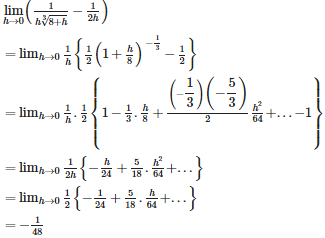# Continuity and Differentiability - Online Test

Q1.  is equal to
Explaination / Solution:

Q2. is equal to
Explaination / Solution:

Q3.  is equal to
Explaination / Solution:

Q4. If [x] stands for the integral part of x, then
Explaination / Solution:

The is defined as the value which takes the nearest small integer in every integer interval . For example in the interval  the value of the function is 0 since it is the lowest integer in that interval . Hence which gives the value as 1,which is the lowest integer of that interval.

Q5.  is equal to
Explaination / Solution:

limxπ2[sinx]=0[sinceπ2hxπ2+h]

Q6. limx01cosxx2 is equal to
Explaination / Solution:

(UsingL’hospital Rule ).

Q7.  is equal to
Explaination / Solution:

Q8. is equal to
Explaination / Solution:Q9. If k be an integer, then  (x –[x])
Explaination / Solution:

= k - (k - 1) = 1 for all
Q10.

is equal to

Explaination / Solution:

( using L’Hospital Rule)